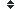half (75 synonym found)

### Sort half by:    Alphabetically| Length| Random | DefinitionDefinition of half:

(adj) : consisting of a moiety, or half; consisting of some indefinite portion resembling a half; approximately a half, whether more or less; partial; imperfect
"a half bushel; a half hour; a half dollar; a half view"
(v) : in an equal part or degree; in some part approximating a half; partially; imperfectly; to halve
"half-colored; half done; half-hearted; half persuaded; half conscious"
(n) : One of two equal parts into which anything may be divided, or considered as divided; -- sometimes followed by of; as, a half of an apple; half of a standard measure; frequently used for half a pint of beer or cider
"three-quarters minus a quarter is a half."
History
This word has been
viewed 2,460 times.

Related searches
for half:

Most Recent

Most Popular

Definition of half:

(adj) : consisting of a moiety, or half; consisting of some indefinite portion resembling a half; approximately a half, whether more or less; partial; imperfect
"a half bushel; a half hour; a half dollar; a half view"
(v) : in an equal part or degree; in some part approximating a half; partially; imperfectly; to halve
"half-colored; half done; half-hearted; half persuaded; half conscious"
(n) : One of two equal parts into which anything may be divided, or considered as divided; -- sometimes followed by of; as, a half of an apple; half of a standard measure; frequently used for half a pint of beer or cider
"three-quarters minus a quarter is a half."